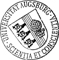## Full-counting statistics of time-dependent conductors

• We develop a scheme for the computation of the full-counting statistics of transport described by Markovian master equations with an arbitrary time dependence. It is based on a hierarchy of generalized density operators, where the trace of each operator yields one cumulant. This direct relation offers a better numerical efficiency than the equivalent number-resolved master equation. The proposed method is particularly useful for conductors with an elaborate time dependence stemming, e.g., from pulses or combinations of slow and fast parameter switching. As a test bench for the evaluation of the numerical stability, we consider time-independent problems for which the full-counting statistics can be computed by other means. As applications, we study cumulants of higher order for two time-dependent transport problems of recent interest, namely steady-state coherent transfer by adiabatic passage (CTAP) and Landau-Zener-Stückelberg-Majorana (LZSM) interference in an open double quantumWe develop a scheme for the computation of the full-counting statistics of transport described by Markovian master equations with an arbitrary time dependence. It is based on a hierarchy of generalized density operators, where the trace of each operator yields one cumulant. This direct relation offers a better numerical efficiency than the equivalent number-resolved master equation. The proposed method is particularly useful for conductors with an elaborate time dependence stemming, e.g., from pulses or combinations of slow and fast parameter switching. As a test bench for the evaluation of the numerical stability, we consider time-independent problems for which the full-counting statistics can be computed by other means. As applications, we study cumulants of higher order for two time-dependent transport problems of recent interest, namely steady-state coherent transfer by adiabatic passage (CTAP) and Landau-Zener-Stückelberg-Majorana (LZSM) interference in an open double quantum dot.• 104288.pdfAuthor: Mónica BenitoORCiDGND, Michael Niklas, Sigmund Kohler urn:nbn:de:bvb:384-opus4-1042883 https://opus.bibliothek.uni-augsburg.de/opus4/104288 2469-9950OPAC Physical Review B American Physical Society (APS) College Park, MD Article English 2016 Universität Augsburg 2023/05/10 94 195433 https://doi.org/10.1103/PhysRevB.94.195433 Mathematisch-Naturwissenschaftlich-Technische Fakultät Mathematisch-Naturwissenschaftlich-Technische Fakultät / Institut für Physik Mathematisch-Naturwissenschaftlich-Technische Fakultät / Institut für Physik / Professur für Quantencomputing und Quantengeräte 5 Naturwissenschaften und Mathematik / 53 Physik / 530 PhysikDeutsches Urheberrecht